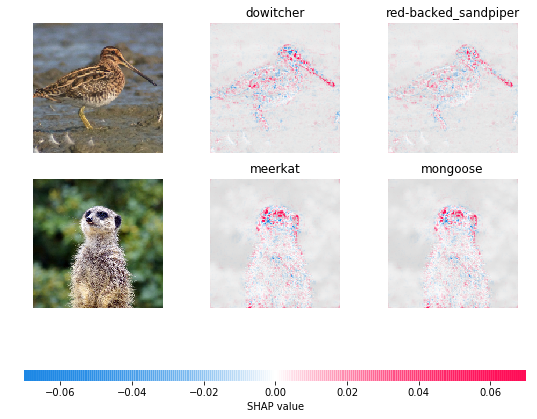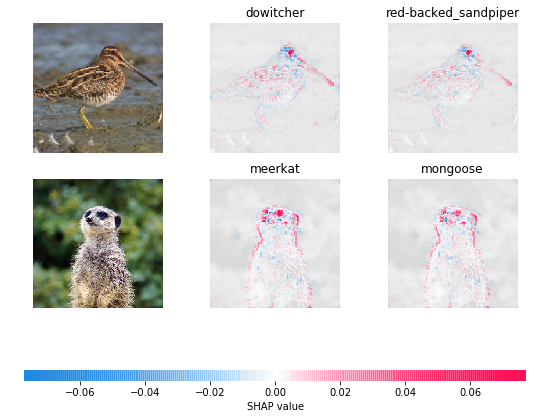# Explain an Intermediate Layer of VGG16 on ImageNet (PyTorch)

Explaining a prediction in terms of the original input image is harder than explaining the predicition in terms of a higher convolutional layer (because the higher convolutional layer is closer to the output). This notebook gives a simple example of how to use GradientExplainer to do explain a model output with respect to the 7th layer of the pretrained VGG16 network.

Note that by default 200 samples are taken to compute the expectation. To run faster you can lower the number of samples per explanation.

:

import torch, torchvision
from torch import nn
from torchvision import transforms, models, datasets
import shap
import json
import numpy as np

:

mean = [0.485, 0.456, 0.406]
std = [0.229, 0.224, 0.225]

def normalize(image):
if image.max() > 1:
image /= 255
image = (image - mean) / std
# in addition, roll the axis so that they suit pytorch

:

# load the model
model = models.vgg16(pretrained=True).eval()

X,y = shap.datasets.imagenet50()

X /= 255

to_explain = X[[39, 41]]

# load the ImageNet class names
url = "https://s3.amazonaws.com/deep-learning-models/image-models/imagenet_class_index.json"
fname = shap.datasets.cache(url)
with open(fname) as f:

shap_values,indexes = e.shap_values(normalize(to_explain), ranked_outputs=2, nsamples=200)

# get the names for the classes
index_names = np.vectorize(lambda x: class_names[str(x)])(indexes)

# plot the explanations
shap_values = [np.swapaxes(np.swapaxes(s, 2, 3), 1, -1) for s in shap_values]

shap.image_plot(shap_values, to_explain, index_names)## Explain with local smoothing

Gradient explainer uses expected gradients, which merges ideas from integrated gradients, SHAP, and SmoothGrad into a single expection equation. To use smoothing like SmoothGrad just set the local_smoothing parameter to something non-zero. This will add normally distributed noise with that standard deviation to the input during the expectation calculation. It can create smoother feature attributions that better capture correlated regions of the image.

:

# note that because the inputs are scaled to be between 0 and 1, the local smoothing also has to be
# scaled compared to the Keras model
explainer = shap.GradientExplainer((model, model.features), normalize(X), local_smoothing=0.5)
shap_values,indexes = explainer.shap_values(normalize(to_explain), ranked_outputs=2, nsamples=200)

# get the names for the classes
index_names = np.vectorize(lambda x: class_names[str(x)])(indexes)

# plot the explanations
shap_values = [np.swapaxes(np.swapaxes(s, 2, 3), 1, -1) for s in shap_values]

shap.image_plot(shap_values, to_explain, index_names)